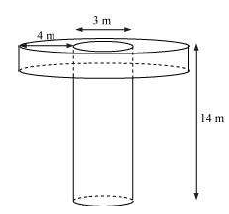# A well of diameter 3 m is dug 14 m deep. The earth taken out of it has been spread evenly all around it in the shape of a circular ring of width 4 m to form an embankment. Find the height of the embankment.Given

Diameter of cylinder = 3 m

Radius of cylinder (r) = 3/2m

Height of cylinder (h) = 14 m

Width of embankment = 4 m

Outer Radius of embankment R1= radius of cylinder + width = 3/2 + 4 = 11/2m

Find out

We have to find the the height of the embankment

Solution

Volume of Embankment = Volume of Cylinder

(πR12H) – (πR22H) = πr2h

(R1–R22)H = (3/2)2× 14

H = $$\frac{(\frac{3}{2})^{2}\times 14}{(\frac{11}{2})^{2}-(\frac{3}{2})^{2}}$$

H = $$\frac{\frac{63}{2}}{(\frac{121}{4})-(\frac{9}{4})}$$

H = $$\frac{\frac{63}{2}}{28}$$

H = $$\frac{63}{228}$$

H = $$\frac{9}{8}$$ H = 1.125 m

Hence, the height of the embankment = 1.125 m